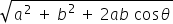science
Easy

Question

# Parallelogram law is used when _______

## Two tails of the vectors are connectedTwo heads of the vectors are connectedVectors are not connectedNone of the aboveHint:

## The correct answer is: Two tails of the vectors are connected

### Parallelogram law is used when two tails of the vectors are connected. Two vectors have the same initial point hence, they have the same initial point.

In parallelogram law of vector addition, it says that if we place two vectors so they have the same initial points(the tails are connected) and then complete the vectors into a parallelogram, then the sum of the vectors is directed diagonal that starts at the same point as the vectors.

science

science

science

### Two vectors started from the origin and moved to the east and north direction 10 m, 15 m, respectively, and the angle between the vectors is 30 degrees, then find the resultant of the vectors.

If two vectors A and B with magnitude a and b respectively are given and the angle between them is θ Then the magnitude of the Resultant of A and B is R =### Two vectors started from the origin and moved to the east and north direction 10 m, 15 m, respectively, and the angle between the vectors is 30 degrees, then find the resultant of the vectors.

If two vectors A and B with magnitude a and b respectively are given and the angle between them is θ Then the magnitude of the Resultant of A and B is R =science

science

science

### Find the magnitude of vectors whose displacement is 15 m and 25 m, with the angle between them being 120 degrees.science

science

science

### If a vector quantity is divided by the scalar, then the whole quantity becomesscience

science

science

### Pythagoras theorem is used inscience

science

### If the vectors are of the same magnitude and same direction, they are said to be

Two vectors are said to be equal if they have same magnitude and direction, regardless of the positions of their positions of their initial points.

### If the vectors are of the same magnitude and same direction, they are said to be

Two vectors are said to be equal if they have same magnitude and direction, regardless of the positions of their positions of their initial points.

science

### The angle between the vectors is _______ if they are orthogonal

Two vectors are orthogonal when they are perpendicular to each other. i.e. 90 degrees and the dot product of the two vectors is 0.

### The angle between the vectors is _______ if they are orthogonal

Two vectors are orthogonal when they are perpendicular to each other. i.e. 90 degrees and the dot product of the two vectors is 0.#### With Turito Foundation.#### Get an Expert Advice From Turito.